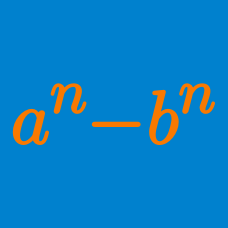Algebra

Factorization of Cubics

If $a$, $b$ and $c$ are real numbers such that $a+b+c=0, abc=-14,$ what is the value of $a^2(b+c)+b^2(c+a)+c^2(a+b)?$

For a linear expression $f(x)=ax+b$, the polynomial $g(x) = x^3-x^2+2f(x)$ can be factorized as $(x-1)(x+\alpha)(x+\beta)$. If $\alpha\beta=22$, what is the value of $f(12)$?

$x$ is a number such that $x^2+5x+25=0$. What is the value of $x^3$?

What is the largest positive integer $a$ such that the cubic polynomial $x^3-21x^2+(20+a)x-a$ can be factorized as the product of $3$ distinct linear polynomials?

If $a$ is a real number and a cubic equation $f(x) = x^3-18x^2+(a+32)x-2a$ has a repeated root, what is the sum of all the possible values of $a$?

×